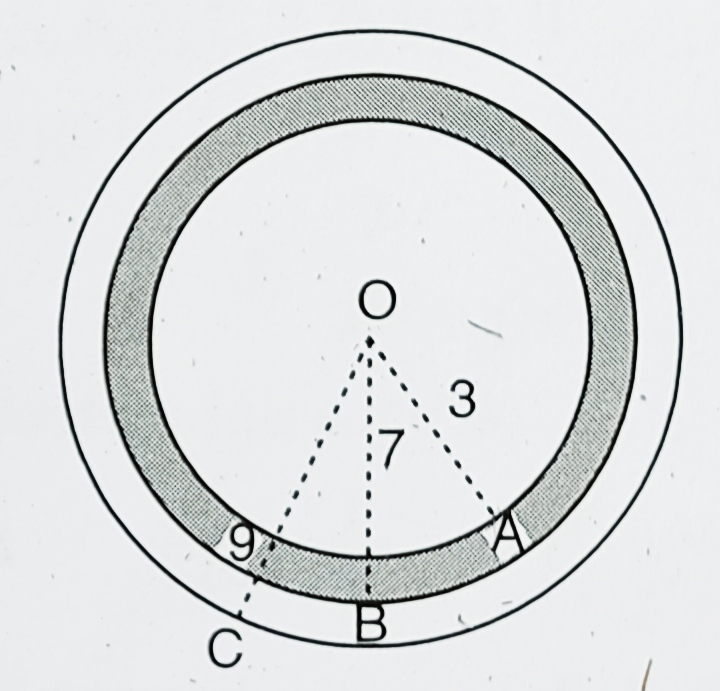"
">

# A target shown in the figure consists of three concentric circles of radii 3, 7 and 9 cm respectively. A dart is thrown and lands on the target. What is the probability that the dart will land on the shaded region?"

Given:

A target shown in the figure consists of three concentric circles of radii 3, 7 and 9 cm respectively.

A dart is thrown and lands on the target.

To do:

We have to find the probability that the dart will land on the shaded region.

Solution:

From the figure,

$\mathrm{OA}=3 \mathrm{~cm}, \mathrm{OB}=7 \mathrm{~cm}, \mathrm{OC}=9 \mathrm{~cm}$

We know that,

Area of a circle of radius $r=\pi r^2$.

Area of the circle with radius $\mathrm{OC}=\pi(9)^{2}$

$=81 \pi$
Area of the shaded region $=\pi(\mathrm{OB})^{2}-\pi(\mathrm{OA})^{2}$

$=\pi(7)^{2}-\pi(3)^{2}$

$=49 \pi-9 \pi$

$=40 \pi$

Therefore,
Probability that the dart will land on the shaded region $=\frac{\text { Area of the shaded region } }{\text { Area of the outer circle }}$

$=\frac{40 \pi}{81 \pi}$

$=\frac{40}{81}$

The probability that the dart will land on the shaded region is $\frac{40}{81}$.

Updated on: 10-Oct-2022

117 Views